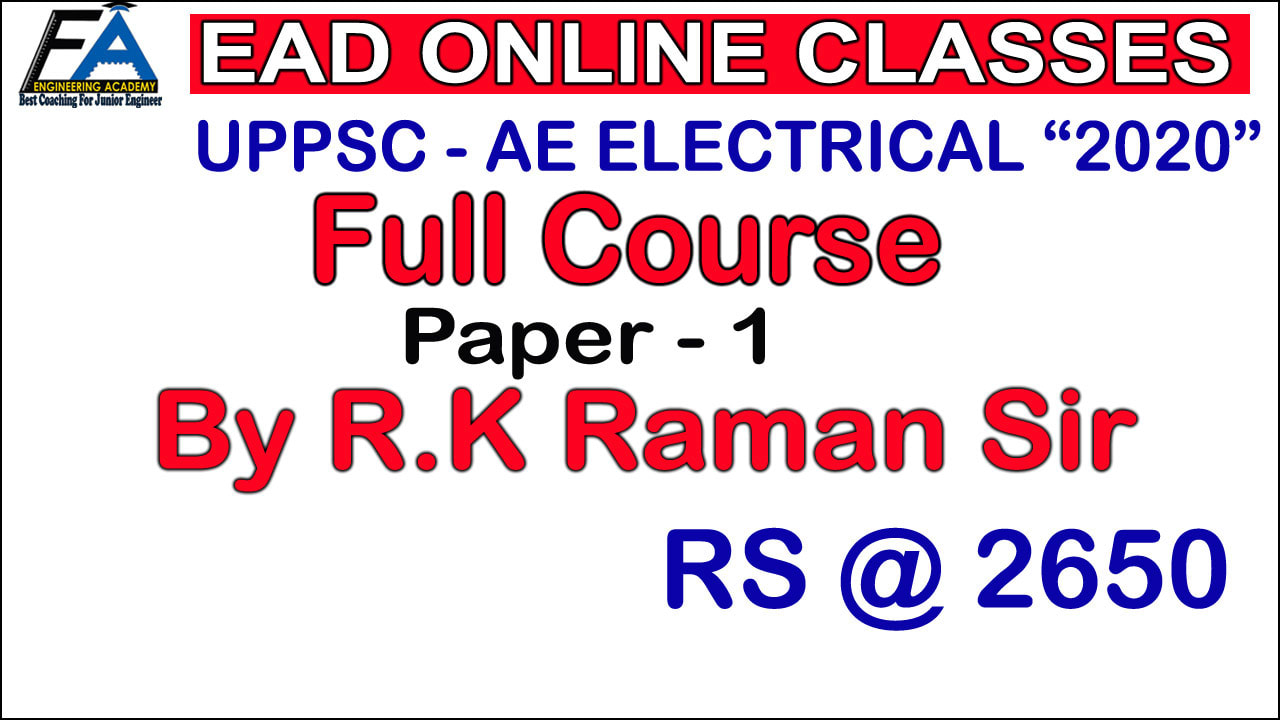HELPLINE NUMBER 8057871234 TOPIC COVERED IN THIS COURSE PAPER-I ELECTRICAL ENGINEERING PAPER-I-100 Questions-300 Marks 1. Networks and Systems: Steady-state and Transient-state Analysis of systems, Thevenin's-, Norton's-, Superposition- and Maximum Power Transfer-theorems, Driving point Transfer functions, Two-port networks, Laplace and Fourier transforms and their applications in Network analysis, Z-transforms for discrete systems, R-L, R-C & L-C network synthesis. 2. Electromagnetic Field Theory: Analysis of electrostatic and magnetostatic fields, Laplace, Poission and Maxwell equations, solution of boundary value problems, electromagnetic wave propagation, ground and space waves, Propagation between Earth Station and Satellites. 3. Control System: Mathematical modelling of dynamic linear continuous systems, Block diagrams and Signal flow graphs, time-response specifications, steady-state error, RouthHurwitz criterion, Nyquist techniques, Root Loci, Bode Plots, Polar Plot, and stability analysis, Lag-, Lead-, Lag-Lead-compensation, state-space modelling, state transition matrix, controllability and observability. 4. Elements of Electronics: Basics of semiconductor diodes, BJT, FET and their chracteristics, different types of transistors and FET amplifiers equivalent circuits and frequency response, feedback oscillators, colpitts oscillator and Hartley Oscillator, Operational amplifiers-chracteristics and applications. 5. Power System Analysis and Design: Line parameters and calculations, Performance of Transmission lines, Mechanical design of overhead lines and Insulators, Corona and radio interference Parameters of single- and three-core Cables, Bus admittance matrix, Load flow equations and methods of solutions, Fast-decoupled load flow, Balance- and Unbalanced-faults analysis, Power system stability, Power system transients and travailing Waves, EHV Transmission, HVDC transmission, Concepts of FACTS, Voltage Control and Economic operation, Concepts of distributed generation, solar and wind power, smart grid concepts. 6. Elements of Electrical Machines: General concepts of E.m.f., m.m.f., and torque in rotating machines, DC Machines: motor and generator characteristics, equivalent circuits, commutation and amature reaction, starting and speed controls of motors; Synchronous Machines: performance, regulation, Parallel operation of generators, motor starting, characteristics and applications, Transformers: phasor-diagram and equivalent circuit, efficiency, and voltage regulation, auto-transformers, 3-phase transformers. 7. Measurement: Basic methods of measurement, Precision and standards, error analysis, Bridges and Poteniometers; moving coil, Moving iron, dynamometer and induction type instruments, measurement of voltage, current, power, energy, and power factor, Instrument transformers, digital voltmeters and multimeters, phase-, time- and frequencymeasurement, Q-meters Oscilloscopes, Basics of sensors, and data acquisition system, Instrumentation systems for pressure and temperature measurements. NON TECH-25 QUESTIONS-75 MARKS GENERAL HINDI